# obw

Occupied bandwidth

## Syntax

``bw = obw(x)``
``bw = obw(x,fs)``
``bw = obw(pxx,f)``
``bw = obw(sxx,f,rbw)``
``bw = obw(___,freqlims,p)``
``````[bw,flo,fhi,power] = obw(___)``````
``obw(___)``

## Description

````bw = obw(x)` returns the 99% occupied bandwidth `bw` of the input signal `x`.```

example

````bw = obw(x,fs)` returns the occupied bandwidth in terms of the sample rate `fs`.```

example

````bw = obw(pxx,f)` returns the 99% occupied bandwidth of the power spectral density (PSD) estimate `pxx`. The frequencies `f` correspond to the estimates in `pxx`.```
````bw = obw(sxx,f,rbw)` computes the occupied bandwidth of the power spectrum estimate `sxx`. The frequencies `f` correspond to the estimates in `sxx`. The function uses the resolution bandwidth `rbw` to integrate each power estimate.```
````bw = obw(___,freqlims,p)` specifies the frequency interval over which to compute the occupied bandwidth. This syntax can include any combination of input arguments from previous syntaxes, as long as the second input argument is either `fs` or `f`. If the second input is passed as empty, `obw` assumes a normalized frequency. This syntax also specifies `p` as the percentage of the total signal power contained in the occupied band.```

example

``````[bw,flo,fhi,power] = obw(___)``` also returns the lower and upper bounds of the occupied bandwidth and the occupied band power.```
````obw(___)` with no output arguments plots the PSD or power spectrum in the current figure window and annotates the bandwidth.```

## Examples

collapse all

Generate 1024 samples of a chirp sampled at 1024 kHz. The chirp has an initial frequency of 50 kHz and reaches 100 kHz at the end of the sampling. Add white Gaussian noise such that the signal-to-noise ratio is 40 dB. Reset the random number generator for reproducible results.

```nSamp = 1024; Fs = 1024e3; SNR = 40; rng default t = (0:nSamp-1)'/Fs; x = chirp(t,50e3,nSamp/Fs,100e3); x = x+randn(size(x))*std(x)/db2mag(SNR);```

Estimate the occupied bandwidth of the signal and annotate it on a plot of the power spectral density (PSD).

`obw(x,Fs)````ans = 5.5377e+04 ```

Generate another chirp. Specify an initial frequency of 200 kHz, a final frequency of 300 kHz, and an amplitude that is twice that of the first signal. Add white Gaussian noise.

```x2 = 2*chirp(t,200e3,nSamp/Fs,300e3); x2 = x2+randn(size(x2))*std(x2)/db2mag(SNR);```

Concatenate the chirps to produce a two-channel signal. Estimate the occupied bandwidth of each channel.

`y = obw([x x2],Fs)`
```y = 1×2 105 × 0.5538 1.0546 ```

Annotate the occupied bandwidths of the two channels on a plot of the PSDs.

`obw([x x2],Fs);`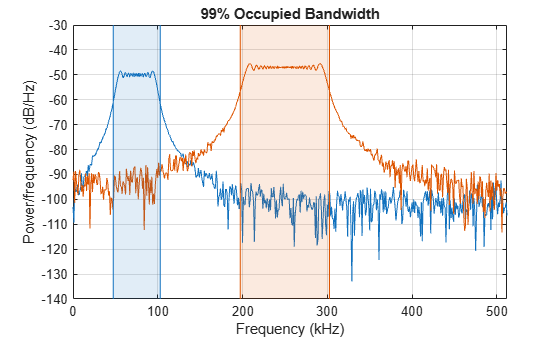Add the two channels to form a new signal. Plot the PSD and annotate the occupied bandwidth.

`obw(x+x2,Fs);`Generate 1024 samples of a 100.123 kHz sinusoid sampled at 1024 kHz. Add white Gaussian noise such that the signal-to-noise ratio is 40 dB. Reset the random number generator for reproducible results.

```nSamp = 1024; Fs = 1024e3; SNR = 40; rng default t = (0:nSamp-1)'/Fs; x = sin(2*pi*t*100.123e3); x = x + randn(size(x))*std(x)/db2mag(SNR);```

Use the `periodogram` function to compute the power spectral density (PSD) of the signal. Specify a Kaiser window with the same length as the signal and a shape factor of 38. Estimate the occupied bandwidth of the signal and annotate it on a plot of the PSD.

```[Pxx,f] = periodogram(x,kaiser(nSamp,38),[],Fs); obw(Pxx,f);```Generate another sinusoid, this one with a frequency of 257.321 kHz and an amplitude that is twice that of the first sinusoid. Add white Gaussian noise.

```x2 = 2*sin(2*pi*t*257.321e3); x2 = x2 + randn(size(x2))*std(x2)/db2mag(SNR);```

Concatenate the sinusoids to produce a two-channel signal. Estimate the PSD of each channel and use the result to determine the occupied bandwidth.

```[Pyy,f] = periodogram([x x2],kaiser(nSamp,38),[],Fs); y = obw(Pyy,f)```
```y = 1×2 103 × 7.2001 7.3777 ```

Annotate the occupied bandwidths of the two channels on a plot of the PSDs.

`obw(Pyy,f);`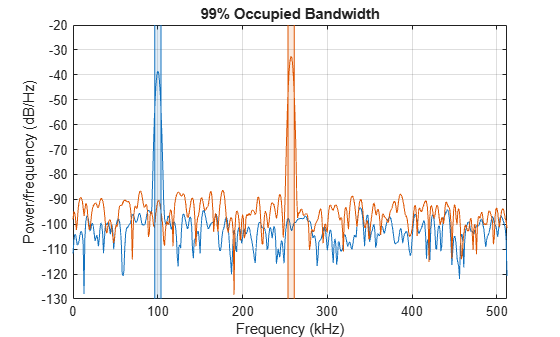Add the two channels to form a new signal. Estimate the PSD and annotate the occupied bandwidth.

```[Pzz,f] = periodogram(x+x2,kaiser(nSamp,38),[],Fs); obw(Pzz,f);```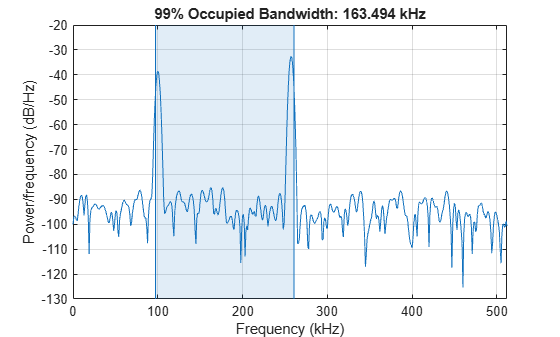Generate a signal whose PSD resembles the frequency response of an 88th-order bandpass FIR filter with normalized cutoff frequencies $0.25\pi$ rad/sample and $0.45\pi$ rad/sample.

`d = fir1(88,[0.25 0.45]);`

Compute the 99% occupied bandwidth of the signal between $0.2\pi$ rad/sample and $0.6\pi$ rad/sample. Plot the PSD and annotate the occupied bandwidth and measurement interval.

`obw(d,[],[0.2 0.6]*pi);`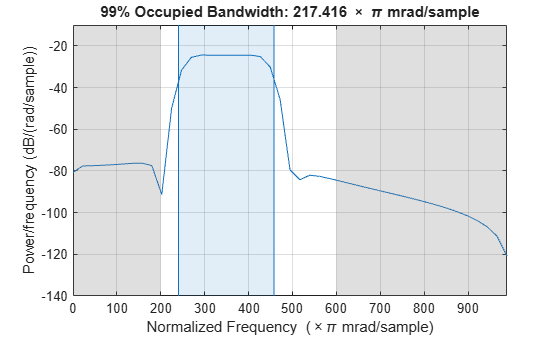Output the occupied bandwidth, its lower and upper bounds, and the occupied band power. Specifying a sample rate of $2\pi$ is equivalent to leaving the rate unset.

```[bw,flo,fhi,power] = obw(d,2*pi,[0.2 0.6]*pi); fprintf('bw = %.3f*pi, flo = %.3f*pi, fhi = %.3f*pi \n',[bw flo fhi]/pi)```
```bw = 0.217*pi, flo = 0.240*pi, fhi = 0.458*pi ```
`fprintf('power = %.1f%% of total',power/bandpower(d)*100)`
```power = 99.0% of total ```

Add a second channel with normalized cutoff frequencies $0.5\pi$ rad/sample and $0.8\pi$ rad/sample and an amplitude that is one-tenth that of the first channel.

`d = [d;fir1(88,[0.5 0.8])/10]';`

Compute the 50% occupied bandwidth of the signal between $0.3\pi$ rad/sample and $0.9\pi$ rad/sample. Plot the PSD and annotate the occupied bandwidth and measurement interval.

`obw(d,[],[0.3 0.9]*pi,50);`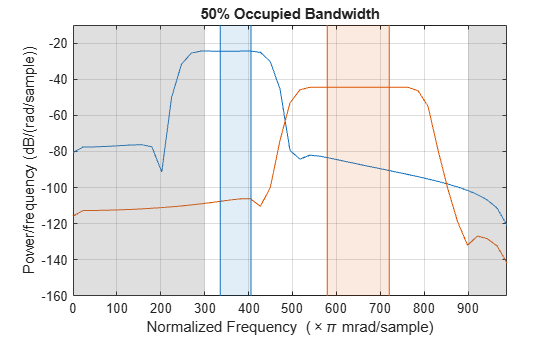Output the occupied bandwidth of each channel. Divide by $\pi$.

`bw = obw(d,[],[0.3 0.9]*pi,50)/pi`
```bw = 1×2 0.0705 0.1412 ```

## Input Arguments

collapse all

Input signal, specified as a vector or matrix. If `x` is a vector, it is treated as a single channel. If `x` is a matrix, then `obw` computes the occupied bandwidth independently for each column. `x` must be finite-valued.

Example: `cos(pi/4*(0:159))+randn(1,160)` is a single-channel row-vector signal.

Example: `cos(pi./[4;2]*(0:159))'+randn(160,2)` is a two-channel signal.

Data Types: `single` | `double`

Sample rate, specified as a positive real scalar. The sample rate is the number of samples per unit time. If the time is measured in seconds, then the sample rate is in hertz.

Data Types: `single` | `double`

Power spectral density (PSD), specified as a vector or matrix with real nonnegative elements. If `pxx` is a one-sided estimate, then it must correspond to a real signal. If `pxx` is a matrix, then `obw` computes the occupied bandwidth of each column of `pxx` independently.

The power spectral density must be expressed in linear units, not decibels. Use `db2pow` to convert decibel values to power values.

Example: ```[pxx,f] = periodogram(cos(pi./[4;2]*(0:159))'+randn(160,2))``` specifies the periodogram PSD estimate of a noisy two-channel sinusoid sampled at 2π Hz and the frequencies at which it is computed.

Data Types: `single` | `double`

Frequencies, specified as a vector.

Data Types: `single` | `double`

Power spectrum estimate, specified as a vector or matrix with real nonnegative elements. If `sxx` is a matrix, then `obw` computes the occupied bandwidth of each column of `sxx` independently.

The power spectrum must be expressed in linear units, not decibels. Use `db2pow` to convert decibel values to power values.

Example: `[sxx,w] = periodogram(cos(pi./[4;2]*(0:159))'+randn(160,2),"power")` specifies the periodogram power spectrum estimate of a two-channel sinusoid embedded in white Gaussian noise and the normalized frequencies at which it is computed.

Data Types: `single` | `double`

Resolution bandwidth, specified as a positive scalar. The resolution bandwidth is the product of two values: the frequency resolution of the discrete Fourier transform and the equivalent noise bandwidth of the window used to compute the PSD.

Data Types: `single` | `double`

Frequency limits, specified as a two-element vector of real values. If you do not specify `freqlims`, then `obw` uses the entire bandwidth of the input signal.

Data Types: `single` | `double`

Power percentage, specified as a positive scalar between 0 and 100. `obw` computes the difference in frequency between the points where the integrated power crosses the ½(100 – `p`) and ½(100 + `p`) percentages of the total power in the spectrum.

Data Types: `single` | `double`

## Output Arguments

collapse all

Occupied bandwidth, returned as a scalar or vector.

• If you specify a sample rate, then `bw` has the same units as `fs`.

• If you do not specify a sample rate, then `bw` has units of rad/sample.

Bandwidth frequency bounds, returned as scalars or vectors.

Power stored in bandwidth, returned as a scalar or vector.

## Algorithms

To determine the occupied bandwidth, `obw` computes a periodogram power spectral density estimate using a rectangular window and integrates the estimate using the midpoint rule. The occupied bandwidth is the difference in frequency between the points where the integrated power crosses 0.5% and 99.5% of the total power in the spectrum.

## Version History

Introduced in R2015a

expand all